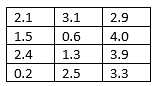# Single-Sample, Confidence Interval for a Mean using t-distribution

The grade point averages for 12 randomly selected students are shown in the table below. Find the 99% confidence interval around the population mean, µ. Assume the population is normally distributed.Solution:

This is a small sample, n < 30, and we do not know sigma. Although small samples generally are t-distributions, the deciding factor for BUS233 is the lack of the population standard deviation, sigma. Therefore, use the t-distribution.

The Excel solution is:Rounding to two decimal places, the interval is (1.22, 3.41).

For the StatCrunch solution, use the Stat > T Stats > One Sample > With Data sequence to open the dialog box.

Select the column containing the data, click on the radio button next to Confidence Interval for µ, enter the confidence level, c, and click Compute!Again, we get the interval (1.22, 3.41).This site uses Akismet to reduce spam. Learn how your comment data is processed.